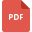# mathematical analysis tutorialsGeometry, Grade 8, India, Mathematical Analysis, Mathematical EconomicsIGCSE Math 2019
Mathematical Logic, England, Mathematical Analysis, Fundamental Math or Basic MathTriangles and its all properties
Mathematical Analysis, Grade 7, India, Geometric MeasurementFractions Assessment (non-calculator)
Mathematical Analysis, Grade 5, India, Fractions and Division, MathematicsMathematical AnalysisBasic introduction of vectors in Math
Mathematical Analysis, United States, Vector, Fundamental Math or Basic MathYear 8 Maths Practice Test Papers
Mathematical Analysis, Grade 8, United KingdomRoyal-Russell-School-Sample-Year-7-Maths-Practice-Paper
Basic Math, Grade 7, United Kingdom, Applied Mathematics, Mathematical Analysis, Mathematical LogicSignificant Figures: Sample Problems with Solutions
Mathematics, Grade 8, United States, Mathematical AnalysisTrigonometry
Mathematical Analysis, India, Trigonometry, Basic Math, Fundamental Math or Basic MathLinear and Nonlinear equations worksheet
Fundamental Math or Basic Math, Grade 8, India, Mathematical Analysis, Mathematical Logic, Linear Equationschange the subject of the formula worksheet
Mathematical Analysis, United States, Mathematical Logicarithmetic ks1 sats
Mathematical Analysis, United Kingdom